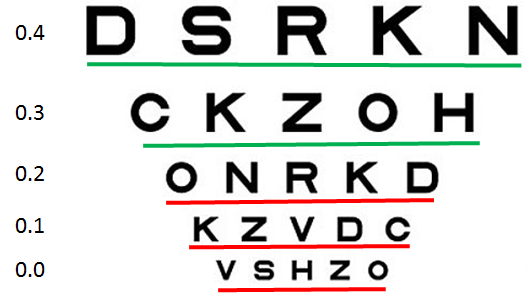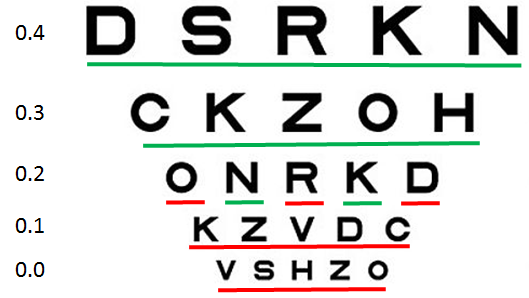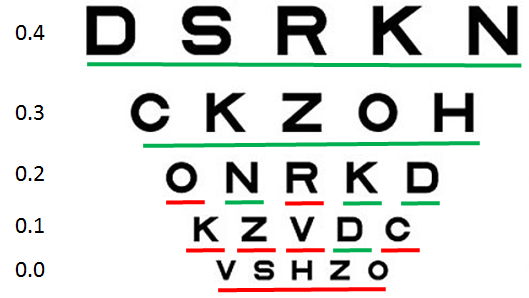The term LogMAR is an acronym for the Logarithm of the Minimum Angle of Resolution.  LogMAR charts have a number of advantages over Snellen charts and have become the gold standard method for assessing visual acuity.

The letter size is described in LogMAR units where LogMAR 0.00 is equivalent to 6/6 (20/20) and LogMAR 1.00 is equivalent to 6/60 (20/200).  The letter size change in units of 0.1 LogMAR from one row to the next.

The Thomson Paediatric Screening Test Chart displays letters from 0.80 LogMAR (largest) to -0.30 LogMAR (smallest) although this range can be set from the Program Options.

LogMAR Scoring simply involves recording the LogMAR size of the smallest letters that can be read.  However, to make things slightly more complicated, patients seldom read all of one row and none of the letters on the row below.  To make scoring rather more precise, LogMAR charts are scored on a "by letter" basis which means that each letter read contributes to the final score.  As the Thomson Paediatric Screening Test Chart displays four letters per row, each letter has a score of 0.025 (4 x 0.025 = 0.10).

This is easier to understand by example.  Consider the chart below: The LogMAR size is shown down the left margin.

In this case the patient read all the letters on the 0.4 and 0.3 rows and none of the letters on the rows below.In this case, their LogMAR score would be simply 0.300.

However, if the patient read the N and the K on the row below:We would need to subtract 2*0.025 =0.05  from the score giving an LogMAR score of 0.250

This applies to any row on the chart so in the final example the patient reads the N K and D on the 0.2 row and the D on the 0.1 row.To calculate the score, take the row number where they got all four correct (0.3), count the number of letters below that that they got correct (4) and multiply this by 0.025 = 0.10.  This is then subtracted from 0.23 to give the LogMAR score of 0.20.

If you are new to LogMAR scoring, helps is at hand in the form of our LogMAR scoring utility, built into the program.